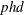### Example 30.3 Variable Selection

This example demonstrates two algorithms of automatic variable selection in the TCOUNTREG procedure. This method is most effective when the number of possible candidates for explaining the variation of some variable is large. For clarity of exposition, this example uses only a small number of variables. The data set ARTICLE published by Long (1997) contains six variables. This data set was already used in ZIP and ZINB Models for Data Exhibiting Extra Zeros. The dependent variable called `art` records the number of articles published by a graduate student in the last three years of their program. Explanatory factors include sex of a student (`fem`), his or her marital status (`mar`), number of children (`kid5`), prestige of the program (`phd`), and publishing activity of the academic adviser (`ment`). All these variables intuitively suggest their affect on students’ primary academic output.

First, for comparison purposes, estimate the simple Poisson model. The choice of model is specified by DIST= option in the MODEL statement.

```proc tcountreg data = long97data;
model art = fem mar kid5 phd ment / dist = poisson;
run;
```

The output of these statements is shown in Output 30.3.1.

Output 30.3.1: Poisson Model for the Number of Published Articles

The TCOUNTREG Procedure

Model Fit Summary
Dependent Variable art
Number of Observations 915
Data Set WORK.LONG97DATA
Model Poisson
Optimization Method Newton-Raphson
Log Likelihood -1651
Number of Iterations 5
AIC 3314
SBC 3343

 Algorithm converged.

Parameter Estimates
Parameter DF Estimate Standard Error t Value Approx
Pr > |t|
Intercept 1 0.304617 0.102982 2.96 0.0031
fem 1 -0.224594 0.054614 -4.11 <.0001
mar 1 0.155243 0.061375 2.53 0.0114
kid5 1 -0.184883 0.040127 -4.61 <.0001
phd 1 0.012823 0.026397 0.49 0.6271
ment 1 0.025543 0.002006 12.73 <.0001

Note that the Newton-Raphson optimization algorithm took five steps to converge. All parameters, except for one, are significant at a 1% or 5% level, while `phd` is not significant even at the 10% level.

In this case, it might be easy to identify variables with limited explanatory power. However, if the number of variables were large, the manual selection could be time demanding and inaccurate. For a large number of variables, you would be better off by applying one of the automatic algorithms of variable selection. The following statements use the penalized likelihood method, which is indicated by SELECT=PEN option in the MODEL statement:

```proc tcountreg data = long97data method = qn;
model art = fem mar kid5 phd ment / dist   = poisson
select = PEN;
run;
```

The output of these statements is shown in Output 30.3.2.

Output 30.3.2: Poisson Model for the Number of Published Articles with Penalized Likelihood Method

The TCOUNTREG Procedure

Model Fit Summary
Dependent Variable art
Number of Observations 915
Data Set WORK.LONG97DATA
Model Poisson
Optimization Method Quasi-Newton
Log Likelihood -1651
Number of Iterations 7
AIC 3312
SBC 3336

 Algorithm converged.

Parameter Estimates
Parameter DF Estimate Standard Error t Value Approx
Pr > |t|
Intercept 1 0.345174 0.060125 5.74 <.0001
fem 1 -0.225303 0.054615 -4.13 <.0001
mar 1 0.152175 0.061067 2.49 0.0127
kid5 1 -0.184993 0.040139 -4.61 <.0001
ment 1 0.025761 0.001950 13.21 <.0001

The Parameter Estimates table shows that the variablewas dropped from the model.

The next statements use the information criterion by specifying the SELECT=INFO option.The direction of search is chosen to be FORWARD, and the information criterion is AIC. In order to achieve the same selection of variables as for the penalized likelihood method, 0.001 is specified for the percentage of decrease in the information criterion necessary for the algorithm to stop.

```proc tcountreg data = long97data;
model art = fem mar kid5 phd ment / dist      = poisson
select    = INFO
( direction = forward
criter    = AIC
lstop     = 0.001 );
run;
```

The output of these statements is shown in Output 30.3.3.

Output 30.3.3: Poisson Model for the Number of Published Articles with Search Method Using Information Criterion

The TCOUNTREG Procedure

Variable Selection Information
Step Effect Entered Effect Removed AIC SBC
0 Base Model   3487.146950 3491.965874
1 ment   3341.286487 3350.924335
2 fem   3330.744604 3345.201376
3 kid5   3316.593036 3335.868733
4 mar   3312.348824 3336.443445

Model Fit Summary
Dependent Variable art
Number of Observations 915
Data Set WORK.LONG97DATA
Model Poisson
Optimization Method Newton-Raphson
Log Likelihood -1651
Number of Iterations 0
AIC 3312
SBC 3336

 Algorithm converged.

Parameter Estimates
Parameter DF Estimate Standard Error t Value Approx
Pr > |t|
Intercept 1 0.345174 0.060125 5.74 <.0001
fem 1 -0.225303 0.054615 -4.13 <.0001
mar 1 0.152175 0.061067 2.49 0.0127
kid5 1 -0.184993 0.040139 -4.61 <.0001
ment 1 0.025761 0.001950 13.21 <.0001

From the output, it is clear that the same set of variables was chosen as the result of information criterion algorithm. Note that the forward optimization algorithm starts with the constant as the only explanatory variable.